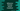# 11 differences between Python 2 vs Python 3 with examples### Introduction:

This is the first question that comes to everyone’s mind when starting development with Python. Python 2 was released 2000 and Python 3 was in 2008. Python 3 is the latest python version and this was started to fix all problems in Python 2. Python 3 is backward incompatible.

The development of Python 2 was stopped in 2020 and it was discontinued. So, if you are just starting Python, you can start with Python 3. In this post, I will discuss on these two versions, which one to choose for learning and the differences between Python 2 and Python 3.

### Python 2 or Python 3:

For someone who is starting to learn Python, Python 3 is the version to start with. Because,

• Most libraries are in Python 3 or slowly all projects are migrating to Python 3.
• Python 3 is easy to learn and the syntax is easy to understand than Python 2.
• The community support for Python 3 is better than Python 2.
• If you want to learn machine learning, data science or AI, you need to learn Python 3.

### Difference between Python 2 vs Python 3:

We learned the basic differences between Python 2 and Python 3 and I hope that you got an idea on which one to pick to learn.

Let’s take a look at the main differences between these versions with examples:

1. Python 2 started in 2000 and python 3 in 2008. Python 2 is discontinued and it will no longer be maintained starting from 2020.

2. Python 3 is not backward compatible. We can port a project from Python 2 to Python 3. But, Python 3 to Python 2 is not possible.

3. The syntax of Python 3 is simpler and easy to understand, but the syntax of Python 2 is difficult to understand.

4. If we divide two integers in Python 2, we will get an integer. But, if we divide two integers in Python 3, we will get a float. For example:

``print(10/3)``

It will give:

``````\$ python2 example.py
3
\$ python3 example.py
3.3333333333333335
\$``````
1. The string is stored as Unicode in Python 3. But, string type is ASCII in Python 2. For example,
``````h = 'hello'
h1 = u'hello'

print(type(h))
print(type(h1))``````

It will print:

``````\$ python2 example.py
<type 'str'>
<type 'unicode'>
\$ python3 example.py
<class 'str'>
<class 'str'>``````

We have to use u to create an unicode string in Python 2.

1. print is different in both Python 2 and Python 3. print is a keyword in Python 2 which is replaced by print() function in Python 3. In Python 2, you have to use:
``print 'hello world'``

In Python 3, you have to use:

``print('hello world')``
1. xrange is no longer available in Python 3. We have to use range function. Both are different. xrange returns a xrange object, but range returns a list.
``````for i in xrange(3):
print i``````

If you run this in Python 2, it will print:

``````0
1
2``````

If you want to write this function in Python 3, it will be as like below:

``````for i in range(3):
print(i)``````

It will give the same result.

1. Raising an exception is different in Python 2 and Python 3. In python 2, we can raise an exception as like:
``raise NameError, 'name error'``

But, in Python 3, we have to enclose the arguments in parenthesis:

``raise NameError('name error')``
1. Handling exception is also different in Python 2 and Python 3. We have to use ‘as’ to handle exceptions in Python 3. For example, in Python 2, we can handle an exception as like below:
``````try:
blah
except NameError, e:
print e, 'exception !!'``````

It will print:

``name 'blah' is not defined exception !!``

In Python 3, it will be as like below:

``````try:
blah
except NameError as e:
print(e, 'exception !!')``````
1. Global variables might change if we use them inside another loop in Python 2. In Python 3, they never change. For example,
``````i = 10
print(i)

[i for i in range(5)]

print(i)``````

If I run this in Python 2, it will print:

``````10
4``````

The value of i changed as we used it in the loop. But, if we have to use it in Python 3,

``````i = 10
print(i)

[i for i in range(5)]

print(i)``````

It will print:

``````10
10``````
1. The input function reads the data as int in Python 2. This reads as a string in Python 3.
``````v = input('Enter a value: ')

print(type(v))``````

It will give:

``````\$ python2 example.py
Enter a value: 123
<type 'int'>
\$ python3 example.py
Enter a value: 123
<class 'str'>``````

We have to use raw_input in Python 2 to read the input as a string.